# JP4591565B2 - Reception device, wireless communication system, position estimation method, and program - Google Patents

Reception device, wireless communication system, position estimation method, and program Download PDF

## Info

Publication number
JP4591565B2
JP4591565B2 JP2008182961A JP2008182961A JP4591565B2 JP 4591565 B2 JP4591565 B2 JP 4591565B2 JP 2008182961 A JP2008182961 A JP 2008182961A JP 2008182961 A JP2008182961 A JP 2008182961A JP 4591565 B2 JP4591565 B2 JP 4591565B2
Authority
JP
Japan
Prior art keywords
phase
receiving
antennas
plurality
Prior art date
Legal status (The legal status is an assumption and is not a legal conclusion. Google has not performed a legal analysis and makes no representation as to the accuracy of the status listed.)
Active
Application number
JP2008182961A
Other languages
Japanese (ja)
Other versions
JP2010019803A (en
Inventor

Original Assignee
ソニー株式会社
Priority date (The priority date is an assumption and is not a legal conclusion. Google has not performed a legal analysis and makes no representation as to the accuracy of the date listed.)
Filing date
Publication date
Application filed by ソニー株式会社 filed Critical ソニー株式会社
Priority to JP2008182961A priority Critical patent/JP4591565B2/en
Publication of JP2010019803A publication Critical patent/JP2010019803A/en
Application granted granted Critical
Publication of JP4591565B2 publication Critical patent/JP4591565B2/en
Application status is Active legal-status Critical
Anticipated expiration legal-status Critical

## Images

•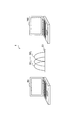•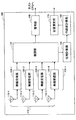•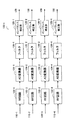•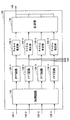•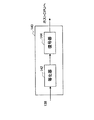•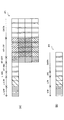•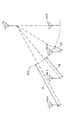•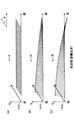•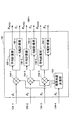•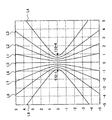•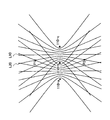•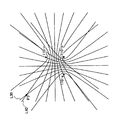•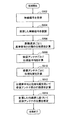•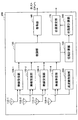•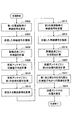•••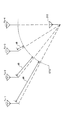•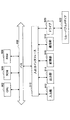## Classifications

• GPHYSICS
• G01MEASURING; TESTING
• G01S3/00Direction-finders for determining the direction from which infrasonic, sonic, ultrasonic, or electromagnetic waves, or particle emission, not having a directional significance, are being received
• G01S3/02Direction-finders for determining the direction from which infrasonic, sonic, ultrasonic, or electromagnetic waves, or particle emission, not having a directional significance, are being received using radio waves
• G01S3/14Systems for determining direction or deviation from predetermined direction
• G01S3/46Systems for determining direction or deviation from predetermined direction using antennas spaced apart and measuring phase or time difference between signals therefrom, i.e. path-difference systems
• G01S3/48Systems for determining direction or deviation from predetermined direction using antennas spaced apart and measuring phase or time difference between signals therefrom, i.e. path-difference systems the waves arriving at the antennas being continuous or intermittent and the phase difference of signals derived therefrom being measured
• HELECTRICITY
• H04ELECTRIC COMMUNICATION TECHNIQUE
• H04BTRANSMISSION
• H04B7/02Diversity systems; Multi-antenna system, i.e. transmission or reception using multiple antennas
• H04B7/04Diversity systems; Multi-antenna system, i.e. transmission or reception using multiple antennas using two or more spaced independent antennas
• H04B7/08Diversity systems; Multi-antenna system, i.e. transmission or reception using multiple antennas using two or more spaced independent antennas at the receiving station
• H04B7/0837Diversity systems; Multi-antenna system, i.e. transmission or reception using multiple antennas using two or more spaced independent antennas at the receiving station using pre-detection combining
• H04B7/0842Weighted combining
• H04B7/086Weighted combining using weights depending on external parameters, e.g. direction of arrival [DOA], predetermined weights or beamforming
• HELECTRICITY
• H04ELECTRIC COMMUNICATION TECHNIQUE
• H04LTRANSMISSION OF DIGITAL INFORMATION, e.g. TELEGRAPHIC COMMUNICATION
• H04L27/00Modulated-carrier systems
• H04L27/26Systems using multi-frequency codes
• H04L27/2601Multicarrier modulation systems
• H04L27/2647Arrangements specific to the receiver
• GPHYSICS
• G01MEASURING; TESTING
• G01S3/00Direction-finders for determining the direction from which infrasonic, sonic, ultrasonic, or electromagnetic waves, or particle emission, not having a directional significance, are being received
• G01S3/02Direction-finders for determining the direction from which infrasonic, sonic, ultrasonic, or electromagnetic waves, or particle emission, not having a directional significance, are being received using radio waves
• G01S3/14Systems for determining direction or deviation from predetermined direction
• G01S3/46Systems for determining direction or deviation from predetermined direction using antennas spaced apart and measuring phase or time difference between signals therefrom, i.e. path-difference systems
• GPHYSICS
• G01MEASURING; TESTING
• G01S5/00Position-fixing by co-ordinating two or more direction or position line determinations; Position-fixing by co-ordinating two or more distance determinations
• G01S5/02Position-fixing by co-ordinating two or more direction or position line determinations; Position-fixing by co-ordinating two or more distance determinations using radio waves
• G01S5/06Position of source determined by co-ordinating a plurality of position lines defined by path-difference measurements
• HELECTRICITY
• H04ELECTRIC COMMUNICATION TECHNIQUE
• H04LTRANSMISSION OF DIGITAL INFORMATION, e.g. TELEGRAPHIC COMMUNICATION
• H04L27/00Modulated-carrier systems
• H04L27/0014Carrier regulation
• H04L2027/0044Control loops for carrier regulation
• H04L2027/0063Elements of loops
• H04L2027/0067Phase error detectors

## Description

The present invention relates to a receiving apparatus, a wireless communication system, a position estimation method, and a program.

In recent years, methods have been proposed for estimating the position of a communication device and the distance to a communication partner using wireless signals transmitted and received by wireless communication. As a method of estimating the distance to the communication partner using a radio signal, for example, a method of estimating according to the time until the signal transmitted to the communication partner returns and returns, or transmitted from the communication partner There is a method of estimating according to the electric field strength of a signal. In addition, as a method for estimating the position of the communication device, there are a method using a signal from a GPS (Global Positioning System), a method combining the above-described distance measuring method, a three-point positioning method, and the like.

Patent Document 1 below discloses an example of a method for determining a distance according to a turnaround time of a radio signal. The technique disclosed in Patent Document 1 below focuses on synchronization between transmitting and receiving apparatuses, and attempts to realize highly accurate distance measurement by correcting the turnaround time based on a predetermined impulse response.

JP 2006-148457 A

However, for example, a method of calculating or acquiring a distance or position using a signal from GPS cannot be used indoors or underground where radio waves from GPS satellites do not reach. In addition, when the radio wave from the GPS satellite is reflected by a building window or the like, it is no longer possible to calculate or obtain a distance or position with practical accuracy.

For example, in the method of estimating the distance to the communication partner according to the return time, it is necessary to strictly determine and guarantee the time until the communication partner receives the wireless signal and returns. Furthermore, when using a radio signal loopback time by a wireless LAN (Local Area Network), a normal synchronization circuit cannot obtain sufficient resolution due to signal propagation speed and sample clock constraints, so that the resolution can be compensated. This requires processing with a high calculation cost.

Further, for example, in the method of determining the distance according to the electric field strength, there is a problem that the influence received from the variation in the transmission power of the communication partner or the noise of the communication environment increases.

Therefore, the present invention has been made in view of the above problems, and an object of the present invention is to provide a new and improved receiving apparatus capable of improving the accuracy of position estimation using a radio signal. A wireless communication system, a position estimation method, and a program.

In order to solve the above-described problem, according to one aspect of the present invention, a plurality of reception antennas each receiving a radio signal in which subcarriers having different frequencies are multiplexed, and each radio received by the plurality of reception antennas are provided. A phase calculation unit that calculates a phase difference from a reference signal for each of the subcarriers included in the radio signal, and the radio signal is transmitted based on the phase difference calculated by the phase calculation unit. And a path difference calculation unit that calculates a path difference that is a difference in distance from any of the plurality of reception antennas to any two reception antennas.

According to such a configuration, a radio signal in which subcarriers having different frequencies are multiplexed is received by each of the plurality of receiving antennas, and the phase difference between the reference signal and the reference signal is calculated for each subcarrier included in each radio signal. Calculated by the division. Then, based on the phase difference calculated by the phase calculation unit, a path difference that is a difference in distance from the transmitting antenna from which the radio signal is transmitted to any two receiving antennas among the plurality of receiving antennas is calculated as a path difference calculation. Calculated by the division.

Further, the phase calculation unit calculates a phase difference average and a phase gradient for each reception antenna using the phase difference for each subcarrier, and the path difference calculation unit is calculated by the phase calculation unit. The path difference may be calculated based on the phase difference average and the phase gradient.

In addition, the path difference calculation unit calculates the path difference for two or more sets of reception antennas among the plurality of reception antennas, and the reception apparatus calculates the two or more sets of reception antennas by the path difference calculation unit. And a position estimation unit that estimates the position of the transmission antenna using the path difference.

Further, the reference signal may be a radio signal received by one receiving antenna among the plurality of receiving antennas.

The phase calculation unit calculates a complex conjugate value of the reference signal, and multiplies the calculated complex conjugate value of the reference signal by a signal value for each subcarrier included in each radio signal. A phase difference may be calculated.

The path difference calculation unit may calculate the path difference by a subspace method based on the phase difference calculated by the phase calculation unit.

Further, the phase calculation unit calculates, for each radio signal received by the plurality of receiving antennas, the phase difference between the reference signal for each subcarrier included in the radio signal a plurality of times, and The path difference calculation unit may reduce an error included in the path difference by calculating the path difference using a plurality of the phase differences calculated a plurality of times by the phase calculation unit.

The receiving apparatus further includes a control unit that controls a frequency band of radio signals received by the plurality of receiving antennas, and the plurality of receiving antennas have different frequencies according to control by the control unit. A radio signal in the first frequency band and a radio signal in the second frequency band, each of which is multiplexed with subcarriers, are received, and the path difference calculation unit is calculated for the radio signal in the first frequency band Using the first phase gradient for each of the reception antennas and the second phase gradient for each of the reception antennas calculated for the radio signal in the second frequency band, the phase gradient used for the calculation of the path difference The error may be reduced.

The radio signal may be a radio signal modulated according to an orthogonal frequency division multiplexing scheme.

In order to solve the above problems, according to another aspect of the present invention, a plurality of radio signals respectively transmitted from a plurality of transmission antennas, each receiving a radio signal in which subcarriers having different frequencies are multiplexed, are received. A reception antenna, a phase calculation unit that calculates a phase difference between each radio signal received by the reception antenna and a reference signal for each subcarrier included in the radio signal, and a calculation by the phase calculation unit A path difference calculating unit that calculates a path difference that is a difference in distance from any two transmitting antennas to the receiving antenna among the plurality of transmitting antennas to which the radio signal has been transmitted, based on the phase difference that has been transmitted; Are provided.

Further, the phase calculation unit calculates a phase difference average and a phase gradient for each of the transmission antennas using the phase difference for each of the subcarriers between the reference signal and the path difference calculation unit, The path difference may be calculated based on the phase difference average and the phase gradient.

In addition, the path difference calculation unit calculates the path difference for two or more sets of transmission antennas among the plurality of transmission antennas, and the receiving device is calculated for the two or more sets of transmission antennas by the path difference calculation unit. In addition, a position estimation unit that estimates the position of the own apparatus using the path difference may be further provided.

Further, the position estimation unit may further estimate the absolute position of the own apparatus using information on the absolute positions of the plurality of transmission antennas acquired from the data part of the radio signal.

In order to solve the above-described problem, according to another aspect of the present invention, a transmission apparatus including a plurality of transmission antennas each transmitting a radio signal in which subcarriers having different frequencies are multiplexed; A receiving antenna that receives a plurality of radio signals transmitted from a transmitting antenna, and calculates a phase difference between each radio signal received by the receiving antenna and a reference signal for each subcarrier included in the radio signal Based on the phase difference calculated by the phase calculation unit and the phase calculation unit, a difference in distance from any two transmission antennas to the reception antenna among the plurality of transmission antennas to which the radio signal is transmitted There is provided a wireless communication system including a receiving device including a path difference calculating unit that calculates a path difference.

In addition, the path difference calculation unit of the reception device calculates the path difference for two or more sets of transmission antennas among the plurality of transmission antennas, and the reception device transmits two or more sets of transmissions by the path difference calculation unit. You may further provide the position estimation part which estimates the relative position of an own apparatus using the said path difference calculated about the antenna.

In addition, the transmission device transmits information on absolute positions of the plurality of transmission antennas to the reception device using the radio signal, and the position estimation unit of the reception device acquires the plurality of information acquired from the radio signal. You may estimate the absolute position of an own apparatus using the information regarding the said absolute position of a transmitting antenna.

In order to solve the above-described problem, according to another aspect of the present invention, a step of receiving wireless signals multiplexed with subcarriers having different frequencies by a plurality of reception antennas, and a plurality of reception antennas, For each received radio signal, a step of calculating a phase difference from a reference signal for each of the subcarriers included in the radio signal, and the radio signal is transmitted based on the calculated phase difference Calculating a path difference that is a difference in distance from a transmitting antenna to any two receiving antennas of the plurality of receiving antennas.

In order to solve the above problems, according to another aspect of the present invention, there is provided a computer for controlling a receiving apparatus having a plurality of receiving antennas that respectively receive radio signals multiplexed with subcarriers having different frequencies. For each radio signal received by the plurality of receiving antennas, a phase calculation unit that calculates a phase difference between each subcarrier included in the radio signal and a reference signal, and the phase calculation unit calculates the phase difference Based on the phase difference, it functions as a path difference calculation unit that calculates a path difference that is a difference in distance from the transmitting antenna from which the radio signal is transmitted to any two receiving antennas among the plurality of receiving antennas. A program is provided.

As described above, according to the receiving apparatus, radio communication system, position estimation method, and program according to the present invention, it is possible to improve the accuracy of position estimation using radio signals.

Exemplary embodiments of the present invention will be described below in detail with reference to the accompanying drawings. In addition, in this specification and drawing, about the component which has the substantially same function structure, duplication description is abbreviate | omitted by attaching | subjecting the same code | symbol.

The “best mode for carrying out the invention” will be described in the following order.
 Overview of Wireless Communication System  First Embodiment (Example of Position Estimation Using Phase Difference Average and Phase Gradient)
 Second embodiment (an example using a plurality of frequency channels)
 Third embodiment (an example of notifying the position from the transmission side to the reception side)

 Overview of Radio Communication System First, an overview of a radio communication system 1 according to an embodiment of the present invention will be described with reference to FIG.

FIG. 1 is a schematic diagram schematically showing a wireless communication system 1 according to an embodiment of the present invention. Referring to FIG. 1, the wireless communication system 1 includes a transmission device 50 and a reception device 100.

Although FIG. 1 shows a PC (Personal Computer) as the transmission device 50 and the reception device 100, the transmission device 50 and the reception device 100 are not limited to an information processing device such as a PC. For example, the transmission device 50 or the reception device 100 is a mobile phone terminal, a PDA (Personal Digital Assistants), a terminal device such as a game terminal, a communication dedicated device such as a wireless access point or a router, or a wireless communication module connected to these devices. It may be.

For example, the transmitter 50 and the receiver 100 are connected by wireless communication according to an OFDM (Orthogonal Frequency Division Multiplexing) scheme. The OFDM system is a modulation system that performs modulation by using sub-carriers (Sub-Carriers) of a plurality of frequencies that are orthogonal to each other in parallel, and is based on standard specifications such as IEEE802.11a, g, n, and wireless USB. It has been adopted.

In the example of FIG. 1, a carrier wave C <b> 1 is shown between the transmission device 50 and the reception device 100. A plurality of subcarriers SC 1 to SC i having different frequencies are multiplexed on the carrier C 1 . The receiving apparatus 100 receives a radio signal transmitted using such a carrier wave C1, and estimates the position of the transmitting apparatus 50.

Note that the wireless communication scheme between the transmission device 50 and the reception device 100 is not limited to the OFDM scheme. Each embodiment described in this specification may be generally applied to multicarrier wireless communication using a plurality of subcarriers having different frequencies.

 First embodiment (an example of position estimation using phase difference average and phase gradient)
Next, a first embodiment of the present invention will be described with reference to FIGS.

FIG. 2 is a block diagram illustrating a logical configuration of the receiving device 100 according to the first embodiment. Referring to FIG. 2, the receiving apparatus 100 includes four receiving antennas 110-n (n = 1 to 4), four wireless receiving units 120-n (n = 1 to 4), a demodulating unit 130, and a decoding unit 140. A phase calculation unit 150, a path difference calculation unit 160, and a position estimation unit 170.

The reception antennas 110-n are connected to the corresponding radio reception units 120-n to form four reception branches. The radio reception unit 120-n is connected to the demodulation unit 130 in parallel. The demodulator 130 is connected to the decoder 140 and the phase calculator 150. The phase calculation unit 150 is connected to the path difference calculation unit 160. The path difference calculation unit 160 is connected to the position estimation unit 170. The decoding unit 140 and the position estimation unit 170 are connected to, for example, a host CPU (Central Processing Unit), for example.

The reception antenna 110-n receives the radio signal transmitted from the transmission device 50, and outputs the received radio signal to the radio reception unit 120-n of the same reception branch.

The radio reception unit 120-n performs processing such as frequency conversion and filtering on the radio signal output from the reception antenna 110-n of the same reception branch.

FIG. 3 is a block diagram illustrating a detailed configuration of the wireless reception unit 120-n. Referring to FIG. 3, the wireless reception unit 120-n includes an RF circuit 122-n, an AD (analog-digital) converter 124-n, a filter 126-n, and an imbalance correction unit 128-n.

The radio signal output from the receiving antenna 110-n is first amplified and frequency-converted in the RF circuit 122-n. The analog signal output from the RF circuit 122-n is converted into a digital signal by the AD converter 124-n. The received signal converted into the digital signal by the AD converter 124-n is filtered by the filter 126-n. Thereafter, the signal output from the filter 126-n is removed from the influence of IQ imbalance by the imbalance correction unit 128-n and input to the demodulation unit 130.

Returning to FIG. 2, the description of the receiving device 100 is continued. The demodulator 130 demodulates the received signals respectively input from the four reception branches.

FIG. 4 is a block diagram illustrating a detailed configuration of the demodulation unit 130. Referring to FIG. 4, the demodulation unit 130 includes a synchronization circuit 132, an FFT (Fast Fourier Transform) circuit 134-n, a channel estimation unit 136-n, and a synthesis unit 138.

The synchronization circuit 132 detects a packet from the reception signals input from the four reception branches, measures the synchronization timing and the frequency offset, cuts out an OFDM symbol from each reception signal, and corresponding FFT circuit 134-n. Output to. The OFDM symbol output from the synchronization circuit 132 is subjected to fast Fourier transform by the FFT circuit 134-n, and is separated for each subcarrier. The reception signal separated for each subcarrier by the FFT circuit 134-n is synthesized by the synthesis unit 138 according to the signal synthesis ratio determined based on the channel estimation result in the channel estimation unit 136-n. Then, the signal synthesized by the synthesis unit 138 is output to the decoding unit 140 as a demodulated stream signal.

In addition, the received signal separated for each subcarrier by the FFT unit 134-n is input to the phase calculation unit 150 in parallel with being input to the channel estimation unit 136-n. The processing in the phase calculation unit 150 will be described in detail later.

Returning to FIG. 2, the description of the receiving device 100 is continued. The decoding unit 140 decodes the demodulated stream signal input from the demodulation unit 130 as described above.

FIG. 5 is a block diagram illustrating a detailed configuration of the decoding unit 140. Referring to FIG. 5, the decoding unit 140 includes an equalizer 142 and a decoder 144.

The demodulated stream signal input to the decoding unit 140 is digitally demodulated by the equalizer 142 and then decoded by the decoder 144. The decoded data signal is output to the host CPU.

FIG. 6 illustrates an example of a configuration of a packet included in a radio signal that may be transmitted from the transmission device 50 to the reception device 100 in the present embodiment.

FIG. 6A shows an example of the configuration of the packet P1 defined in the IEEE802.11n communication specification employing the OFDM method.

Referring to FIG. 6A, a packet P1 includes an L-STF (Legacy-Short Training Field), an L-LTF (Legacy-Long Training Field), an L-SIG (Legacy-Signal Field), and an HT-SIG (i.e. Throughput-SIG), HT-STF, HT-LTF, and Data (data part) fields are included.

Among these, the L-STF is used for detecting a packet by the above-described synchronization circuit 132, for example. The L-LTF is used for frequency offset measurement by the synchronization circuit 132 and channel estimation by the channel estimation unit 136-n, for example. L-SIG and HT-SIG include control information such as data length, communication rate, and modulation method. The HT-STF is used for gain adjustment, for example, and the HT-LTF is used for acquisition of a MIMO (Multiple Input Multiple Output) channel matrix. The data part includes a data symbol.

On the other hand, FIG. 6B is an example of the configuration of the packet P2 defined in the IEEE802.11a communication specification adopting the OFDM method. Referring to FIG. 6B, packet P2 includes STF, LTF, SIG, and Data fields.

Here, for example, when one radio signal transmitted from one transmission antenna is received using the plurality of reception antennas 110-n, the radio signal is transmitted on the communication path according to the position of the reception antenna 110-n. There is a difference in the route length.

FIG. 7 is an explanatory diagram conceptually showing the difference in the path length of the radio signal according to the position of the receiving antenna 110-n. Referring to FIG. 7, a path of a radio signal from the transmission antenna Tx to the reception antennas 110-1, 110-2, 110-3, and 110-4 is shown.

In FIG. 7, an arc STD indicates a locus of a position having a distance equal to the path length of the radio signal from the transmission antenna Tx to the reception antenna 110-4 between the transmission antenna Tx.

Based on the path length to the receiving antenna 110-4, the path length from the transmitting antenna Tx to the receiving antenna 110-1 is longer by the distance d1. It can also be seen that the path length from the transmission antenna Tx to the reception antenna 110-2 is longer by the distance d2, and the path length from the transmission antenna Tx to the reception antenna 110-3 is longer by the distance d3. In this specification, such differences in path length between receiving antennas, that is, distances d1, d2, d3, for example, are referred to as path differences.

When there is a path difference d between the receiving antennas 110-n as described above, a phase shift occurs in the radio signal received by the receiving antenna 110-n. The phase shift of the radio signal received by the receiving antenna 110-n, that is, the relationship between the phase difference φ between the receiving antennas 110-n and the path difference d is expressed by the following equation.

(1)

Here, λ is the wavelength of the radio signal, and m is an integer indicating how many times the phase has rotated during the path difference d.

According to Expression (1), when the path difference d is constant, the phase difference φ depends on the wavelength λ of the radio signal. Therefore, when, for example, an OFDM radio signal in which sub-carriers having different frequencies are multiplexed is used as a radio signal, the same reception antenna 110-n is received by the reception antenna 110-n. The detected phase differs for each subcarrier of the radio signal.

FIG. 8 is an explanatory diagram conceptually showing the phase difference for each subcarrier in the same receiving antenna 110-n.

8A to 8C, FIG. 8A shows the phase difference for each subcarrier when the path difference d from the reference antenna is d = 0. On the other hand, FIG. 8B shows the phase difference for each subcarrier when the path difference d is medium, and FIG. 8C shows the phase difference for each subcarrier when the path difference d is large.

A number of small arrows shown in FIGS. 8A to 8C represent each subcarrier included in the radio signal. The horizontal axis in each figure corresponds to the frequency of the subcarrier. That is, the arrow shown on the right side in the figure corresponds to a subcarrier having a higher frequency (that is, a shorter wavelength) than the arrow shown on the left side in the figure. The rotation angle of the arrow with respect to the horizontal axis represents the phase difference φ detected for the corresponding subcarrier.

Referring to FIG. 8A, when the path difference d = 0, the phase difference φ is constant regardless of the subcarrier frequency. On the other hand, in the example of FIG. 8B, if the average of the phase difference between the subcarriers is an arrow AVG, the phase difference φ is larger than that of the arrow AVG for the subcarrier having a low frequency and the phase difference φ is for a subcarrier having a high frequency. Is smaller than the arrow AVG. Such a phase twist can be quantified as a change in phase with respect to a predetermined change in frequency. In the present specification, such a phase change amount is referred to as a phase gradient. In the example of FIG. 8C in which the path difference d is larger, the phase twist is further increased.

As an example, in the IEEE 802.11n standard 40 MHz mode, the center frequency of the radio signal frequency band is f mid = 5 [GHz], the lower end frequency f btm = 4.98 [GHz], and the upper end frequency f top = 5. 02 [GHz]. Then, the wavelength λ mid = 6.00 [cm] for the central frequency, the wavelength λ btm = 6.024 [cm] for the lower end frequency, and the wavelength λ top = 5.976 [cm] for the upper end frequency.

Here, it is assumed that the path difference d = 6 [cm]. In that case, the phase difference φ mid = 2π at the center frequency, that is, the average phase difference φ AVG = 2π of all subcarriers in one frequency band. On the other hand, the phase difference φ btm = 2π × (6.00 / 6.024) at the lower end frequency and the phase difference φ top = 2π × (6.00 / 5.976) at the upper end frequency. Therefore, the phase twist between both ends of the frequency band is φ top −φ btm = about 2.88 [deg].

The relationship between the phase difference average φ AVG between the receiving antennas 110-n and the phase gradient θ in the receiving antenna 110-n and the path difference d between the receiving antennas 110-n will be further described.

From equation of the discrete Fourier transform, the relationship between the signal x n + 1 which remained signal x n and one sample is expressed by the following equation.

(2)
(3)
(4)

That is, a phase twist corresponding to one round (360 °) occurs over all subcarriers in one frequency band. The path difference corresponding to the twist between both ends of the 40 [MHz] frequency band is 3 × 10 8 [m] / 40 [MHz] = 7.5 [m]. Therefore, the twist of the phase reaches one round at the path difference d = 7.5 [m]. The path difference d = 6 [cm] corresponds to 2.88 [deg].

Here, if the phase difference average φ AVG between the receiving antennas 110-n is 0 [deg], the path difference d is either 0 or an integer multiple of the wavelength λ, and the phase difference average φ path difference is only the value of AVG d is uniquely determined. Therefore, in the present embodiment, the path difference d is determined in consideration of the phase gradient θ based on the following relational expression.

First, the relationship between the wavelength λ mid of the center frequency (corresponding to the carrier frequency) and the wavelength λ s of an arbitrary subcarrier is derived from the equation (1) as follows:

(5)
(6)

Here, δφ is a phase difference between the phase at the center frequency and the phase at the subcarrier. If the phase difference between adjacent subcarriers on the frequency axis is defined as a phase gradient θ, the phase difference δφ is given by the following equation.

(7)

Here, N is the number of subcarriers existing between the center frequency and the frequency of the subcarrier. The relationship between the wavelength λ mid and the wavelength λ s is expressed by the following equation using the center frequency f mid and the subcarrier frequency f s .

(8)

From the equations (5), (6), and (8), the following equation is derived.

(9)

Further, the phase twist ratio θ λ in the subcarrier is defined as follows.

(10)

Then, the integer m in the relational expression (1) between the path difference d and the phase difference φ is derived from Expression (9) and Expression (10) as follows:

(11)

Of the parameters that determine m in equation (11), θ λ does not depend on the path difference d. Therefore, when the path difference d changes according to the position of the receiving antenna 110-n, the path difference is calculated using the phase difference average φ AVG for each receiving antenna 110-n and the phase gradient θ for determining δφ. It will be appreciated that an integer m can be determined which represents how many phases the phase has d during d.

When the integer m is determined, the path difference d between the receiving antennas 110-n can be calculated by the equation (1) using the wavelength λ mid and the phase difference average φ AVG at the center frequency.

In FIG. 2, the phase calculation unit 150 uses the demodulated stream signal input from the demodulation unit 130 to calculate the phase difference average φ AVG required for calculating the path difference d between the reception antennas 110-n described above. And calculate the phase gradient θ.

FIG. 9 is a block diagram illustrating a detailed configuration of the phase calculation unit 150. Referring to FIG. 9, the phase calculation unit 150 includes a complex conjugate calculation unit 152, a multiplier 154-m (m = 1, 2, 3), an average calculation unit 156-m, and a gradient calculation unit 158-m.

In the example of FIG. 9, the phase calculation unit 150 uses an input signal corresponding to a radio signal received by the reception antenna 110-4 among the reception antennas 110-n as a reference signal for phase difference calculation. Therefore, the stream signal S 4 received and demodulated by the reception antenna 110-4 is input to the complex conjugate calculation unit 152. On the other hand, the stream signals S 1 , S 2 and S 3 received and demodulated by the receiving antennas 110-1, 2 and 3 are input to multipliers 154-1, 154-2 and 154-3, respectively.

Complex conjugate calculation unit 152 calculates the complex conjugate value of the stream signal S 4, and inputs the resulting signal multipliers 154-1 and 154-2, and the 154-3.

Multipliers 154-1 and 154-2, and 154-3 multiplies the complex conjugate value of the stream signal S 4 that is input from the complex conjugate calculation unit 152 and the stream signal S 1, S 2, S 3 and, respectively. As a result, the phase difference between each of the signal values for each subcarrier included in the stream signals S 1 , S 2 , S 3 and the reference signal is calculated.

The average calculation unit 156-1 uses the phase difference of each sub-carrier of the stream signals S 1 calculated by the multiplier 154-1, calculates the phase difference average phi AVG1 the receiving antenna 110-1. Further, the gradient calculation unit 158-1, by using the phase difference, compute the phase gradient theta 1 of the receive antenna 110-1.

Similarly, the average calculation unit 156-2 is a phase difference average phi AVG2 the receiving antenna 110-2, the gradient calculation unit 158-2 calculates a phase gradient theta 2 of the receiving antenna 110-2. Further, the average calculation unit 156-3 calculates the phase difference average φ AVG3 for the reception antenna 110-3, and the gradient calculation unit 158-3 calculates the phase gradient θ 3 for the reception antenna 110-3.

The phase difference average φ AVG1 and phase gradient θ 1 , phase difference average φ AVG2 and phase gradient θ 2 , and phase difference average φ AVG3 and phase gradient θ 3 obtained as a result of these processes are output to the path difference calculation unit 160. The

Here, an example in which the reference signal used as the reference for calculating the phase difference is the signal received by the receiving antenna 110-4 has been described. However, the reference signal may be a signal received by any receiving antenna or a known signal given in advance.

In FIG. 2, the path difference calculation unit 160 uses the phase difference average and the phase gradient for each reception antenna 110-n input from the phase calculation unit 150, according to the above formulas (1) to (11). A path difference from the transmitting antenna to which the signal is transmitted to any two receiving antennas among the receiving antennas 110-n is calculated.

Here, the result of calculation by the path difference calculation unit 160, the path difference d 14 between the receiving antenna 110-1 receiving antenna 110-4 is obtained. Then, the position of the transmission antenna from which the radio signal is transmitted can be considered to be on a curved surface where the difference in distance from the reception antenna 110-1 and the reception antenna 110-4 is constant (ie, d 14 ).

10, in the two-dimensional plane in which the receiving antenna 110-1 through the reception antenna 110-4 is an explanatory view showing the position of the transmitting antennas to be estimated from the path difference d 14.

In FIG. 10, the receiving antenna 110-1 and the receiving antenna 110-4 are located at coordinates (−1, 0) and (1, 0) on the horizontal axis passing through the origin.

When the path difference d 14 between the receiving antenna 110-1 and the receiving antenna 110-4 is d 14 = 0, the transmitting antenna is on a straight line L0 passing through an intermediate point between the receiving antenna 110-1 and the receiving antenna 110-4. Located in.

When the path difference d 14 ≠ 0, the locus drawn by the position of the transmitting antenna logically draws a hyperbola. The receiving antenna 110-1 and the receiving antenna 110-4 are located at the focal point of the hyperbola.

For example, if 20% of the path difference d 14 is the receiving antenna 110-1 and spacing of the receiving antennas 110-4, the transmitting antenna is located on the hyperbola L1 or L6. Of these, by determining whether the phase of the receiving antenna 110-1 is advanced or delayed with respect to the receiving antenna 110-4, it is possible to determine whether the transmitting antenna is located on the hyperbola L1 or L6. .

Similarly, if 40% of the path difference d 14 is the receiving antenna 110-1 and spacing of the receiving antennas 110-4, the transmitting antenna is located on the hyperbola L2 or L7. If the path difference d 14 is 60% of the distance between the receiving antennas 110-1 receive antenna 110-4, the transmitting antenna is located on the hyperbola L3 or L8. Further, if 80% of the path difference d 14 is the receiving antenna 110-1 and spacing of the receiving antennas 110-4, the transmitting antenna is located on the hyperbola L4 or L9.

FIG. 11 shows the position of the transmitting antenna based on the path difference between the receiving antennas 110-a and 110-b and the path difference between the receiving antennas 110-b and 110-c among the receiving antennas 110-n. It is explanatory drawing for demonstrating an example which estimates this.

In FIG. 11, it is assumed that, for example, it is estimated that the transmission antenna is located on the curve L10 based on the path difference between the reception antennas 110-a and 110-b. Further, based on the path difference between the receiving antennas 110-b and 110-c, for example, it is assumed that the transmitting antenna is located on the curve L20. Then, the transmission antenna is estimated to be located on the intersection P1 or P2 of the curve L10 and the curve L20.

FIG. 12 illustrates another example of estimating the position of the transmitting antenna based on the path difference between the receiving antennas 110-a and 110-b and the path difference between the receiving antennas 110b and 110-c. It is explanatory drawing of.

In FIG. 12, suppose that it is estimated that the transmitting antenna is located on the curve L30, for example, based on the path difference between the receiving antennas 110-a and 110-b. Further, based on the path difference between the receiving antennas 110-b and 110-c, for example, it is assumed that the transmitting antenna is located on the curve L40. Then, it is estimated that the transmission antenna is located on the intersection P3 of the curves L30 and L40.

As described with reference to FIGS. 11 and 12, the position of the transmitting antenna on the two-dimensional plane is estimated by using the path difference calculated for each of the two sets of receiving antennas 110-n. Can do. Further, it is understood that the position of the transmission antenna in the three-dimensional space can be estimated by extending such a concept and using the path differences calculated for each of three or more sets of reception antennas 110-n.

In FIG. 2, the position estimation unit 170 uses the path differences calculated for the two or more sets of reception antennas 110-n by the path difference calculation unit 160 in this way, and the position of the transmission antenna to which the radio signal is transmitted. Is estimated.

FIG. 13 is a flowchart illustrating an example of the flow of the position estimation process of the transmission antenna by the reception device 100 according to the present embodiment.

Referring to FIG. 13, first, a plurality of receiving antennas 110-n respectively receive radio signals in which subcarriers having different frequencies are multiplexed (S502).

Next, the reception signal converted into the digital signal by the radio reception unit 120-n is demodulated by the demodulation unit 130 and separated into signals for each subcarrier (S504).

Then, for each signal separated for each subcarrier, the phase difference between the phase calculation unit 150 and the reference signal is calculated (S506). Here, the phase calculation unit 150 uses, for example, a signal received by one of the reception antennas 110-n as a reference signal, and multiplies the complex conjugate value of the reference signal by the signal value for each subcarrier. The phase difference for each subcarrier is calculated. At this time, the reference signal may not be a signal received by one receiving antenna but a known signal given in advance.

Thereafter, the phase calculation unit 150 calculates a phase difference average for each reception antenna 110-n using the phase difference calculated for each subcarrier (S508). Further, the phase calculation unit 150 calculates a phase gradient for each reception antenna 110-n using the phase difference calculated for each subcarrier (S510).

Then, the path difference calculation unit 160 selects an arbitrary one of the reception antennas 110-n from the transmission antenna from which the radio signal is transmitted based on the phase difference average and the phase gradient for each reception antenna calculated by the phase calculation unit 150. The path difference between the two receiving antennas is calculated (S512).

Further, the position estimation unit 170 estimates the position of the transmission antenna using the path difference calculated for the two or more sets of reception antennas by the path difference calculation unit 160 (S514).

Up to this point, the receiving apparatus 100 according to the first embodiment of the present invention has been described with reference to FIGS. According to the present embodiment, radio signals multiplexed with subcarriers with different frequencies are received by a plurality of reception antennas, and based on the phase difference calculated for each subcarrier included in each radio signal, The path difference to any two receive antennas is calculated. Thereby, for example, it is possible to improve the accuracy of position estimation using a radio signal without obtaining additional requirements such as guarantee of the signal folding time from the communication partner.

At this time, the phase difference for each subcarrier may be calculated by, for example, multiplying the complex conjugate value of the reference signal by the signal value for each subcarrier. Further, the path difference from the transmitting antenna to the two receiving antennas may be calculated based on the average phase difference and the phase gradient for each receiving antenna. In such a case, the path difference between the receiving antennas can be calculated at a relatively low calculation cost.

Further, the path difference may be calculated for any two or more sets of receiving antennas. For example, the position of the communication partner on the two-dimensional plane can be estimated by calculating the path difference for two sets of receiving antennas. In addition, the position of the communication partner in the three-dimensional space can be estimated by calculating the path difference for three or more sets of receiving antennas.

In addition, a radio signal received by one receiving antenna among a plurality of receiving antennas may be used as a reference signal serving as a reference for the phase difference for each subcarrier. In such a case, since the phase difference is calculated between the radio signals that have arrived through the same communication environment, the influence of the communication environment on the signal is canceled out and the accuracy of position estimation can be improved.

In the present embodiment, the path difference from the transmitting antenna to the receiving antenna 110-n is calculated using the phase difference average and the phase gradient. However, instead of using the subspace method such as the MUSIC (Multiple Signal Classification) method or the ESPRIT (Estimation of Signal Parameters via Rotational Invariance Techniques) method to the reception antenna 110n difference calculation. Good.

Further, the above-described phase difference calculation processing by the phase calculation unit 150 may be executed a plurality of times for one radio signal, and the path difference between the receiving antennas may be calculated based on the plurality of phase differences obtained as a result. For example, by calculating the path difference based on the average value or the median value of the phase differences calculated a plurality of times for the same subcarrier, errors included in the calculation result of the path difference can be reduced.

 Second embodiment (an example using a plurality of frequency channels)
In the first embodiment of the present invention, the receiving apparatus 100 that receives a radio signal of one frequency channel in which subcarriers having different frequencies are multiplexed by a plurality of antennas and estimates the position of the transmitting antenna has been described. For example, in the description related to FIG. 8, the relationship between the path difference, the phase difference average, and the phase gradient is described by taking one frequency channel of IEEE802.11n with the central frequency f mid as 5 [GHz] as an example. .

Here, the phase difference or phase gradient between the antennas of each subcarrier appears more clearly when the frequency channel bandwidth is wider. Further, the accuracy of the calculated path difference becomes higher as the wavelength of the carrier wave becomes shorter, and the phase difference appears more gradually with respect to the path difference as the wavelength of the carrier wave becomes longer. Therefore, the receiving apparatus 200 according to the second embodiment of the present invention calculates the phase difference while changing the frequency channel to a plurality of frequency bands, and improves the accuracy of the position estimation process.

FIG. 14 is a block diagram showing a logical configuration of the receiving apparatus 200 according to the second embodiment. Referring to FIG. 14 , the receiving apparatus 200 includes four receiving antennas 110-n, four radio receiving units 120-n, a demodulating unit 130, a decoding unit 140, a phase calculating unit 150, a path difference calculating unit 260, a position estimation. Unit 170 and a frequency control unit 280.

The frequency control unit 280 controls the frequency band of the radio signal received by the reception antenna 110-n by outputting a frequency control signal to the radio reception unit 120-n. Then, the receiving antenna 110-n receives, for example, a first frequency band radio signal and a second frequency band radio signal in which subcarriers having different frequencies are multiplexed according to control by the frequency control unit 280, respectively. Receive. The radio signals in the first and second frequency bands are, for example, radio signals of a frequency channel with a center frequency f mid = 5.00 [GHz] and a frequency channel with a center frequency f mid = 5.08 [GHz]. Also good.

When a radio signal in the first frequency band is received by the reception antenna 110-n, the radio signal in the first frequency band is received for each reception antenna 110-n according to the process described in relation to the first embodiment. The first phase gradient is calculated by the phase calculation unit 150. In addition, when a radio signal in the second frequency band is received by the reception antenna 110-n, similarly, a second phase gradient for the radio signal in the second frequency band is calculated by the phase calculation unit 150. .

The path difference calculation unit 260 calculates the path difference between the receiving antennas 110-n after calculating the average of the first phase gradient and the second phase gradient to reduce the phase gradient error, for example. Instead, the path difference calculation unit 260 calculates the integer m in the equation (1) using the equation (11), for example, and calculates from the first phase difference average and the second phase difference average. A new phase gradient may be further used to determine the integer m, thereby increasing the accuracy of the path difference calculation.

FIG. 15 is a flowchart illustrating an example of the flow of the position estimation process of the transmission antenna by the reception device 200 according to the present embodiment.

Referring to FIG. 15, first, a plurality of receiving antennas 110-n receive a radio signal in which subcarriers having different frequencies are multiplexed in the first frequency band (S602).

Next, the reception signal converted into the digital signal by the radio reception unit 120-n is demodulated by the demodulation unit 130 and separated into signals for each subcarrier (S604).

Then, for each signal separated for each subcarrier, the phase difference between the phase calculation unit 150 and the reference signal is calculated (S606).

Thereafter, the phase calculation unit 150 calculates a phase difference average for each reception antenna 110-n using the phase difference calculated for each subcarrier (S608). Further, the phase calculation unit 150 calculates the first phase gradient for each reception antenna 110-n using the phase difference calculated for each subcarrier (S610).

After the calculation of the phase difference average and the phase gradient by the phase calculation unit 150 is completed, the frequency control unit 280 outputs a frequency control signal to the radio reception unit 120-n, and the radio signal received by the reception antenna 110-n. The frequency band is changed from the first frequency band to the second frequency band (S612).

As a result, in the second frequency band, radio signals in which subcarriers having different frequencies are multiplexed are received by the plurality of receiving antennas 110-n (S614), demodulated by the demodulator 130 (S616), and the phase calculator The phase difference from the reference signal is calculated by 150 (S618).

Then, the phase calculation unit 150 calculates the second phase gradient for each reception antenna 110-n using the phase difference calculated for each subcarrier (S620).

Then, the path difference calculation unit 2 60, based on the phase difference means and first and second phase gradient for each receiving antenna is calculated by the phase calculation unit 150, from the transmitting antenna to any two receive antennas A path difference is calculated (S622).

Further, using the calculated path differences for two or more pairs of receiving antennas by the path difference calculation unit 2 60, position of the transmitting antenna is estimated by the position estimating unit 170 (S624).

Up to this point, the reception device 200 according to the second embodiment of the present invention has been described with reference to FIGS. 14 and 15. According to this embodiment, the phase gradient for each subcarrier is calculated for radio signals received in two or more different frequency bands. Thereby, the error of the path difference calculated based on the phase gradient is reduced, and the accuracy of position estimation is improved.

In this embodiment, an example is described in which the path difference from the transmission antenna is calculated based on the first phase gradient calculated in the first frequency band and the second phase gradient calculated in the second frequency band. did. However, instead, the adjacent first frequency band and second frequency band are regarded as one virtual frequency band with an expanded bandwidth, and the one frequency band is calculated from the phase difference for each subcarrier. Two phase gradients may be calculated. For example, if both the first frequency band and the second frequency band have a bandwidth of 40 MHz, based on the calculated phase difference for each subcarrier, a virtual bandwidth having a bandwidth of 80 MHz is obtained. One phase gradient can be calculated for the frequency band. Even in this case, the error of the path difference calculated based on the phase gradient is reduced, and the accuracy of position estimation is improved.

 Third embodiment (an example of notifying the position from the transmission side to the reception side)
In the first and second embodiments, a plurality of receiving antennas are provided in the receiving device, and the position of the transmitting antenna is determined based on the phase difference calculated for the subcarriers included in the radio signals received by the plurality of receiving antennas. Estimated. On the other hand, in this embodiment, the transmission apparatus is provided with a plurality of transmission antennas, the radio signals from the plurality of transmission antennas are received by one reception antenna of the reception apparatus, and the position is estimated based on the same concept. Do.

FIG. 16 is a schematic diagram schematically illustrating the wireless communication system 3 according to the third embodiment. Referring to FIG. 16, the wireless communication system 3 includes a transmission device 52 and a reception device 300.

In FIG. 16, a wireless access point is shown as the transmission device 52, but the transmission device 52 is not limited to a wireless access point. For example, the transmission device 52 is an information processing device such as a PC or a workstation, a terminal device such as a mobile phone terminal or PDA or a game terminal, a dedicated communication device such as a router, or a wireless communication module connected to these devices. May be.

The transmission device 52 and the reception device 300 are connected by, for example, wireless communication according to the OFDM scheme.

In the present embodiment, the transmission device 52 has a plurality of transmission antennas as will be described later. Then, radio signals in which subcarriers having different frequencies are multiplexed are transmitted from the plurality of transmitting antennas to receiving apparatus 300, respectively. The receiving apparatus 300 receives radio signals transmitted from such a plurality of transmitting antennas and performs position estimation.

FIG. 17 is a block diagram illustrating a logical configuration of the receiving device 300 according to the third embodiment. Referring to FIG. 17, the receiving apparatus 300 includes a receiving antenna 310, a radio receiving unit 320, a demodulating unit 330, a decoding unit 340, a phase calculating unit 350, a path difference calculating unit 360, and a position estimating unit 370.

In the present embodiment, the receiving antenna 310 receives radio signals transmitted from a plurality of transmitting antennas.

FIG. 18 is an explanatory diagram conceptually showing how radio signals transmitted from four transmission antennas reach the reception antenna 310 as an example. Referring to FIG. 18, a path of a radio signal from transmission antennas Tx-1, Tx-2, Tx-3, and Tx-4 to reception antenna 310 is shown. These transmission antennas Tx-1, Tx-2, Tx-3, and Tx-4 are provided in a transmission device 52 as shown in FIG. 16, for example.

In FIG. 18, an arc STD indicates a locus of a position having a distance equal to the path length from the transmission antenna Tx-4 to the reception antenna 310 with the reception antenna 310.

For example, the path length from the transmission antenna Tx-4 to the reception antenna 310 is used as a reference. Then, the path difference for the transmission antenna Tx-1 is d4, the path difference for the transmission antenna Tx-2 is d5, and the path difference for the transmission antenna Tx-3 is d6. These path differences can be obtained from the phase difference for each subcarrier included in the radio signal transmitted from each transmitting antenna Tx-n and received by the receiving antenna 310.

Returning to FIG. 17, the wireless reception unit 320 performs amplification, frequency conversion, analog-digital conversion, filtering, imbalance correction, and the like on the wireless signal output from the reception antenna 310.

The demodulator 330 performs packet detection, synchronization timing and frequency offset measurement, OFDM symbol cutout, fast Fourier transform, channel estimation, and the like on the received signal output from the radio receiver 320. The stream signal demodulated by the demodulator 330 is output to the decoder 340 and the phase calculator 350.

The decoding unit 340 performs digital demodulation and decoding of the demodulated stream signal input from the demodulation unit 330. The decoded data signal is output from the decoding unit 340 to, for example, the host CPU.

Further, the phase calculation unit 350, for each radio signal transmitted from the transmission antenna Tx-n, for each subcarrier included in the stream signal demodulated by the demodulation unit 330, a phase difference from an arbitrary reference signal Calculate The calculation of the phase difference may be performed by multiplying the complex conjugate value of the reference signal by the signal value for each subcarrier. Furthermore, the phase calculation unit 350 calculates the average phase difference and the phase gradient for each transmission antenna Tx-n using the calculated phase difference.

Then, the path difference calculation unit 360, based on the phase difference means and the phase gradient for each transmit antenna, which is calculated by the phase calculation unit 3 5 0, any two transmitting antennas Tx-n of the transmission antennas Tx-n To the receiving antenna 310 is calculated. The calculation of the path difference is performed, for example, according to the above-described equations (1) to (11).

Here, even if the path difference from the reception antenna 310 to the transmission antenna Tx-n is obtained, the reception device 300 specifies the position of the transmission / reception device if the mutual positional relationship of the transmission antennas Tx-n is not known. I can't.

Therefore, in the present embodiment, in the transmission device 52, for example, position information of each transmission antenna Tx-n is inserted into the data portion (DATA) of the packet shown in FIG. In receiving apparatus 300, data signal decoded by decoding section 340 is output to position estimating section 370, and position estimating section 370 acquires the position information of each transmitting antenna Tx-n.

For example, it is assumed that the transmitter 52 inserts absolute position information of each transmission antenna Tx-n into the data portion of the packet. In that case, the position estimation unit 370 of the reception device 300 acquires the absolute position information from the data signal decoded by the decoding unit 340. And the position estimation part 370 is based on the acquired absolute position information and the path difference of two or more sets of transmission antennas Tx-n among the transmission antennas Tx-n, by the method described with reference to FIGS. The absolute position of the receiving antenna 310 can be estimated.

For example, as shown in FIG. 16, such a position estimation method is used when the transmitting device 52 is a fixed wireless access point (or a wireless base station) and the receiving device 300 is a movable terminal device. Is particularly effective. In this case, the transmission device 52 may record the absolute position information of the own device in its own storage device, for example, and transmit the absolute position information in a packet to be transmitted to the reception device 300.

So far, the transmission device 52 and the reception device 300 according to the third embodiment of the present invention have been described with reference to FIGS. According to the present embodiment, a radio signal in which subcarriers having different frequencies are multiplexed is transmitted from the plurality of transmission antennas Tx-n provided in the transmission device 52 to the reception device 300. The phase calculation unit 350 of the receiving apparatus 300 calculates a phase difference for each subcarrier between the wireless signals received from the plurality of transmission antennas and the reference signal. Then, the path difference calculation unit 360 calculates a path difference from any two transmission antennas Tx-n to the reception antenna 310 based on the phase difference calculated by the phase calculation unit 350.

At this time, the absolute position information of the transmitting antenna Tx-n is transmitted to the receiving apparatus 300 using, for example, a data part of a radio signal, and is acquired by the position estimating unit 370 of the receiving apparatus 300. Accordingly, the position estimation unit 370 can estimate the absolute position of the reception antenna 310 of the reception device 300 based on the acquired absolute position information and the above-described path difference.

Here, it does not matter whether the series of processing according to the first to third embodiments described in this specification is realized by hardware or software. When a series of processes or a part thereof is executed by software, a program constituting the software is executed by using a computer incorporated in dedicated hardware or a general-purpose computer shown in FIG.

In FIG. 19, a CPU (Central Processing Unit) 902 controls the overall operation of the general-purpose computer. A ROM (Read Only Memory) 904 stores a program or data describing a part or all of a series of processes. A RAM (Random Access Memory) 906 temporarily stores programs and data used by the CPU 902 for arithmetic processing.

The CPU 902, ROM 904, and RAM 906 are connected to each other via a bus 908. Further, an input / output interface 910 is connected to the bus 908.

The input / output interface 910 is an interface for connecting the CPU 902, the ROM 904, and the RAM 906 to the input unit 912, the output unit 914, the storage unit 916, the communication unit 918, and the drive 920.

The input unit 912 receives an instruction or information input from a user via an input device such as a button, switch, lever, mouse, or keyboard. The output unit 914 outputs information to the user via a display device such as a CRT (Cathode Ray Tube), a liquid crystal display, an OLED (Organic Light Emitting Diode), or an audio output device such as a speaker.

The storage unit 916 includes, for example, a hard disk drive or a flash memory, and stores programs, program data, image data, and the like. The communication unit 918 performs communication processing via a network such as a LAN (Local Area Network) or the Internet. The drive 920 is provided in a general-purpose computer as necessary. For example, a removable medium 922 is attached to the drive 920.

When the series of processing according to the first to third embodiments is executed by software, for example, a program stored in the ROM 904, the storage unit 916, or the removable medium 922 shown in FIG. 19 is read into the RAM 906 at the time of execution. And executed by the CPU 902.

As mentioned above, although preferred embodiment of this invention was described referring an accompanying drawing, it cannot be overemphasized that this invention is not limited to the example which concerns. It will be apparent to those skilled in the art that various changes and modifications can be made within the scope of the claims, and these are naturally within the technical scope of the present invention. Understood.

For example, the position estimation process according to the first embodiment described with reference to FIG. 13 is not necessarily performed in the order described in the flowchart. Each processing step may include processing executed in parallel or individually independently.

It is a schematic diagram which shows the radio | wireless communications system which concerns on 1st and 2nd embodiment. It is a block diagram which shows the logical structure of the receiver which concerns on 1st Embodiment. It is a block diagram which shows an example of a structure of the radio | wireless receiving part which concerns on 1st Embodiment. It is a block diagram which shows an example of a structure of the demodulation part which concerns on 1st Embodiment. It is a block diagram which shows an example of a structure of the decoding part which concerns on 1st Embodiment. It is explanatory drawing which shows an example of a structure of a communication packet. It is explanatory drawing which illustrates the path difference from a transmitting antenna to each receiving antenna. It is explanatory drawing which illustrates the phase difference for every subcarrier, and the twist of a phase. It is a block diagram which shows an example of a structure of the phase calculation part which concerns on 1st Embodiment. It is explanatory drawing which shows an example of the position of the transmission antenna estimated based on a path difference. It is explanatory drawing which shows the other example of the position of the transmission antenna estimated based on a path difference. It is explanatory drawing which shows another example of the position of the transmission antenna estimated based on a path difference. It is a flowchart which shows an example of the position estimation process which concerns on 1st Embodiment. It is a block diagram which shows the logical structure of the receiver which concerns on 2nd Embodiment. It is a flowchart which shows an example of the position estimation process which concerns on 2nd Embodiment. It is a schematic diagram which shows the radio | wireless communications system which concerns on 3rd Embodiment. It is a block diagram which shows the logical structure of the receiver which concerns on 3rd Embodiment. It is explanatory drawing which illustrates the path difference from each transmitting antenna to a receiving antenna. And FIG. 11 is a block diagram illustrating a configuration example of a general-purpose computer.

Explanation of symbols

1, 3 Wireless communication system 50, 52 Transmitting device 100, 200, 300 Receiving device 110-n, 310 Receiving antenna 150, 350 Phase calculation unit 160, 260, 360 Path difference calculation unit 170, 370 Position estimation unit 280 Control unit ( Frequency control unit)

## Claims (15)

1. A plurality of receiving antennas each receiving a radio signal in which subcarriers having different frequencies are multiplexed;
Wherein the phase difference between the reference signal calculated with the plurality of the radio signals received by the receiving antenna for each of the subcarriers contained in this radio signal, using the phase difference for each calculated the subcarriers A phase calculator for further calculating a phase difference average and a phase gradient for each of the receiving antennas ;
Based on the phase difference average calculated by the phase calculation unit and the phase gradient, the difference in distance from the transmitting antenna from which the radio signal is transmitted to any two receiving antennas among the plurality of receiving antennas. A path difference calculation unit for calculating a certain path difference;
A receiving device.
2. The path difference calculating unit calculates the path difference for two or more sets of receiving antennas among the plurality of receiving antennas;
A position estimation unit that estimates the position of the transmission antenna using the path difference calculated for two or more sets of reception antennas by the path difference calculation unit;
Further comprising
The receiving device according to claim 1.
3.   The receiving apparatus according to claim 1, wherein the reference signal is a radio signal received by one receiving antenna of the plurality of receiving antennas.
4.   The phase calculation unit calculates a complex conjugate value of the reference signal, and multiplies the calculated complex conjugate value of the reference signal by a signal value for each subcarrier included in each radio signal. The receiving device according to claim 1, wherein
5. The phase calculation unit calculates, for each radio signal received by the plurality of receiving antennas, the phase difference between the reference signal for each subcarrier included in the radio signal a plurality of times ,
Before SL by using a plurality of the phase differences calculated a plurality of times by the phase calculating section, an error included in the path difference which is calculated by the path difference calculation section is reduced,
The receiving device according to claim 1.
A control unit for controlling a frequency band of radio signals received by the plurality of receiving antennas;
Further comprising:
The plurality of receiving antennas receive a radio signal in a first frequency band and a radio signal in a second frequency band each of which is multiplexed with subcarriers having different frequencies according to control by the control unit;
The path difference calculation unit calculates a first phase gradient for each of the reception antennas calculated for the radio signal in the first frequency band and a frequency for each of the reception antennas calculated for a radio signal in the second frequency band. Using a second phase gradient to reduce the phase gradient error used to calculate the path difference;
The receiving device according to claim 1 .
7.   The receiving apparatus according to claim 1, wherein the radio signal is a radio signal modulated according to an orthogonal frequency division multiplexing scheme.
8. A receiving antenna for receiving a plurality of radio signals transmitted from a plurality of transmitting antennas, each of which is a radio signal in which subcarriers having different frequencies are multiplexed;
Wherein the phase difference between the reference signal and calculates information on the radio signal received by the receiving antenna for each of the subcarriers contained in this radio signal, for each of the subcarriers between the calculated and the reference signal A phase calculation unit that further calculates a phase difference average and a phase gradient for each of the transmission antennas using the phase difference ;
Based on the phase difference average calculated by the phase calculator and the phase gradient, a difference in distance from any two transmitting antennas to the receiving antenna among the plurality of transmitting antennas to which the radio signal is transmitted. A path difference calculation unit for calculating a path difference that is;
A receiving device.
9. The path difference calculation unit calculates the path difference for two or more sets of the transmission antennas;
A position estimator for estimating the position of the own apparatus using the path differences calculated for two or more sets of transmission antennas by the path difference calculator;
Further comprising
The receiving device according to claim 8 .
10. The receiving apparatus according to claim 9 , wherein the position estimating unit further estimates the absolute position of the own apparatus using information on the absolute positions of the plurality of transmitting antennas acquired from the data part of the radio signal.
11. A plurality of transmission antennas each transmitting a radio signal in which subcarriers having different frequencies are multiplexed;
A transmission device comprising:
A receiving antenna for receiving a plurality of radio signals transmitted from the plurality of transmitting antennas;
Wherein the phase difference between the reference signal and calculates information on the radio signal received by the receiving antenna for each of the subcarriers contained in this radio signal, for each of the subcarriers between the calculated and the reference signal A phase calculation unit that further calculates a phase difference average and a phase gradient for each of the transmission antennas using the phase difference ;
And based on the phase difference average calculated by the phase calculation unit and the phase gradient, a distance from any two transmission antennas to the reception antenna among the plurality of transmission antennas to which the radio signal is transmitted A path difference calculation unit for calculating a path difference which is a difference between
A receiving device comprising:
A wireless communication system including:
12. The path difference calculation unit of the receiving device calculates the path difference for two or more sets of the transmission antennas;
A position estimator that estimates a relative position of the own apparatus using the path differences calculated for two or more sets of transmission antennas by the path difference calculator;
Further comprising
The wireless communication system according to claim 11 .
13. The transmitting device transmits information on absolute positions of the plurality of transmitting antennas to the receiving device using the radio signal;
The position estimating unit of the receiving apparatus estimates the absolute position of the own apparatus using information on the absolute positions of the plurality of transmitting antennas acquired from the radio signal;
The wireless communication system according to claim 12 .
14. Receiving radio signals multiplexed with subcarriers having different frequencies by a plurality of receiving antennas;
Calculating a phase difference between each radio signal received by the plurality of receiving antennas and a reference signal for each of the subcarriers included in the radio signal;
Calculating a phase difference average and a phase gradient for each of the receiving antennas using the calculated phase difference for each of the subcarriers;
Based on the calculated phase difference average and the phase gradient , a path difference that is a difference in distance from the transmitting antenna from which the radio signal is transmitted to any two receiving antennas among the plurality of receiving antennas is calculated. Step to do;
A position estimation method including:
15. A computer for controlling a receiving apparatus having a plurality of receiving antennas that respectively receive radio signals multiplexed with subcarriers having different frequencies:
Wherein the phase difference between the reference signal calculated with the plurality of the radio signals received by the receiving antenna for each of the subcarriers contained in this radio signal, using the phase difference for each calculated the subcarriers A phase calculator for further calculating a phase difference average and a phase gradient for each of the receiving antennas ;
Based on the phase difference average calculated by the phase calculation unit and the phase gradient, the difference in distance from the transmitting antenna from which the radio signal is transmitted to any two receiving antennas among the plurality of receiving antennas. A path difference calculation unit for calculating a certain path difference;
Program to function as.
JP2008182961A 2008-07-14 2008-07-14 Reception device, wireless communication system, position estimation method, and program Active JP4591565B2 (en)

## Priority Applications (1)

Application Number Priority Date Filing Date Title
JP2008182961A JP4591565B2 (en) 2008-07-14 2008-07-14 Reception device, wireless communication system, position estimation method, and program

## Applications Claiming Priority (2)

Application Number Priority Date Filing Date Title
JP2008182961A JP4591565B2 (en) 2008-07-14 2008-07-14 Reception device, wireless communication system, position estimation method, and program
US12/498,807 US8456362B2 (en) 2008-07-14 2009-07-07 Receiving apparatus, radio communication system, position estimation method and program

## Publications (2)

Publication Number Publication Date
JP2010019803A JP2010019803A (en) 2010-01-28
JP4591565B2 true JP4591565B2 (en) 2010-12-01

# Family

## Family Applications (1)

Application Number Title Priority Date Filing Date
JP2008182961A Active JP4591565B2 (en) 2008-07-14 2008-07-14 Reception device, wireless communication system, position estimation method, and program

## Country Status (2)

US (1) US8456362B2 (en)
JP (1) JP4591565B2 (en)

## Families Citing this family (11)

* Cited by examiner, † Cited by third party
Publication number Priority date Publication date Assignee Title
US8577387B2 (en) * 2009-03-17 2013-11-05 Qualcomm Incorporated Position location using multiple carriers
US8619908B2 (en) * 2009-12-02 2013-12-31 Harris Corporation Wireless ranging system and related methods
JP5208994B2 (en) * 2010-05-14 2013-06-12 中国電力株式会社 Evacuation guidance method and information processing system used for this method
EP2535738B1 (en) * 2011-06-15 2019-08-07 Airbus Defence and Space GmbH Improved antenna beam forming for tracking a transmitter signal
KR20150104346A (en) * 2014-03-05 2015-09-15 서울시립대학교 산학협력단 System and method for calculating arrangement data between devices
JP6381134B2 (en) * 2015-03-31 2018-08-29 三菱重工機械システム株式会社 Radio wave arrival angle detection device, vehicle detection system, radio wave arrival angle detection method, and vehicle false detection prevention method
JP6401671B2 (en) * 2015-07-15 2018-10-10 日本電信電話株式会社 Position detection apparatus and position detection method
CN108027417A (en) 2015-08-14 2018-05-11 索尼移动通讯有限公司 Relative position between determining device
CN105652240A (en) * 2015-12-29 2016-06-08 南京工程学院 Rail transit vehicle door motion track measurement system and rail transit vehicle door motion track measurement method
EP3316534A1 (en) * 2016-10-27 2018-05-02 Fraunhofer Gesellschaft zur Förderung der Angewand Channel estimation of frequency sub bands
US10499363B1 (en) * 2018-09-18 2019-12-03 Qualcomm Incorporated Methods and apparatus for improved accuracy and positioning estimates

## Citations (7)

* Cited by examiner, † Cited by third party
Publication number Priority date Publication date Assignee Title
JPH09257902A (en) * 1996-03-22 1997-10-03 Mitsubishi Electric Corp Bearing detecting device
JP2001201550A (en) * 2000-01-18 2001-07-27 Mitsubishi Electric Corp Incoming wave estimation device
JP2004333252A (en) * 2003-05-06 2004-11-25 Nippon Telegr & Teleph Corp <Ntt> Apparatus and method for inferring position
JP2006023261A (en) * 2003-07-28 2006-01-26 Rcs:Kk Active tag device
JP2006042201A (en) * 2004-07-29 2006-02-09 Advanced Telecommunication Research Institute International Distance measurement system, distance measuring method and communication equipment
JP2007212424A (en) * 2006-01-10 2007-08-23 Mitsubishi Denki Joho Network Kk Position detecting device and position detection program
JP2009287942A (en) * 2008-05-27 2009-12-10 Mitsubishi Electric Corp Direction finder

## Family Cites Families (5)

* Cited by examiner, † Cited by third party
Publication number Priority date Publication date Assignee Title
JPH0569471B2 (en) * 1987-09-08 1993-10-01 Nippon Electric Co
JP4561329B2 (en) 2004-11-18 2010-10-13 ソニー株式会社 Ranging system, transmitting terminal, receiving terminal, ranging method, and computer program
KR100778309B1 (en) * 2005-12-08 2007-11-22 한국전자통신연구원 Apparatus and Method for computing location of a moving beacon using received signal strength and multi-frequencies
KR100904681B1 (en) * 2005-12-15 2009-06-25 한국전자통신연구원 Method and apparatus for transmitter locating using a single receiver
JPWO2008029812A1 (en) * 2006-09-05 2010-01-21 有限会社アール・シー・エス Distance measuring device

## Patent Citations (7)

* Cited by examiner, † Cited by third party
Publication number Priority date Publication date Assignee Title
JPH09257902A (en) * 1996-03-22 1997-10-03 Mitsubishi Electric Corp Bearing detecting device
JP2001201550A (en) * 2000-01-18 2001-07-27 Mitsubishi Electric Corp Incoming wave estimation device
JP2004333252A (en) * 2003-05-06 2004-11-25 Nippon Telegr & Teleph Corp <Ntt> Apparatus and method for inferring position
JP2006023261A (en) * 2003-07-28 2006-01-26 Rcs:Kk Active tag device
JP2006042201A (en) * 2004-07-29 2006-02-09 Advanced Telecommunication Research Institute International Distance measurement system, distance measuring method and communication equipment
JP2007212424A (en) * 2006-01-10 2007-08-23 Mitsubishi Denki Joho Network Kk Position detecting device and position detection program
JP2009287942A (en) * 2008-05-27 2009-12-10 Mitsubishi Electric Corp Direction finder

## Also Published As

Publication number Publication date
JP2010019803A (en) 2010-01-28
US8456362B2 (en) 2013-06-04
US20100009644A1 (en) 2010-01-14

## Similar Documents

Publication Publication Date Title
KR100816174B1 (en) Transmitting method and apparatus
JP4357488B2 (en) Improved frequency offset tracking
JP4161054B2 (en) Digital signal demodulator
US7215636B2 (en) Method of determining training signal in OFDM, and apparatus and method for receiving OFDM signal using the training signal
JP4661938B2 (en) Wireless communication apparatus, wireless communication method, and computer program
US8077696B2 (en) Wireless communication apparatus and wireless communication method
JP4615059B2 (en) Communication device
US20070025236A1 (en) Method and system for performing synchronization in OFDM systems
US7649963B2 (en) Apparatus for estimating and compensating carrier frequency offset and data receiving method in receiver of wireless communication system
US20070230403A1 (en) Start of packet detection for multiple receiver combining and multiple input multiple output radio receivers
JP4562542B2 (en) Calibration method and base station device, terminal device, and radio device using the same
US20050152484A1 (en) Multicarrier receivers and methods for separating transmitted signals in a multiple antenna system
US20030162547A1 (en) Simulcasting OFDM system having mobile station location identification
US20060176802A1 (en) Apparatus and method for compensating for frequency offset in wireless communication system
US10484814B2 (en) Direction finding and FTM positioning in wireless local area networks
TWI247497B (en) Communication system and method for channel estimation and beamforming using a multi-element array antenna
US8605835B2 (en) Receiver device, integrated circuit, receiving method, and receiving program
EP2147566B1 (en) Techniques to enhance location estimation in an ofdma based system
US20070223393A1 (en) Wireless Communication Apparatus and Comunication Quality Estimating Method
US8208522B2 (en) System and methods for receiving OFDM symbols having timing and frequency offsets
US7957474B2 (en) Robust detection of packet types
KR101829403B1 (en) Channel description feedback in a communication system
US8358722B2 (en) Signal processing apparatus, signal processing method, and reception system
US7627047B2 (en) Orthogonal frequency division multiplexing (OFDM) receiver
JP2001345777A (en) Communication system for ofdm and base station and terminal used for it

## Legal Events

Date Code Title Description
A977 Report on retrieval

Free format text: JAPANESE INTERMEDIATE CODE: A971007

Effective date: 20100416

A131 Notification of reasons for refusal

Free format text: JAPANESE INTERMEDIATE CODE: A131

Effective date: 20100427

A521 Written amendment

Free format text: JAPANESE INTERMEDIATE CODE: A523

Effective date: 20100615

TRDD Decision of grant or rejection written
A01 Written decision to grant a patent or to grant a registration (utility model)

Free format text: JAPANESE INTERMEDIATE CODE: A01

Effective date: 20100817

A01 Written decision to grant a patent or to grant a registration (utility model)

Free format text: JAPANESE INTERMEDIATE CODE: A01

FPAY Renewal fee payment (event date is renewal date of database)

Free format text: PAYMENT UNTIL: 20130924

Year of fee payment: 3

A61 First payment of annual fees (during grant procedure)

Free format text: JAPANESE INTERMEDIATE CODE: A61

Effective date: 20100830

R151 Written notification of patent or utility model registration

Ref document number: 4591565

Country of ref document: JP

Free format text: JAPANESE INTERMEDIATE CODE: R151

FPAY Renewal fee payment (event date is renewal date of database)

Free format text: PAYMENT UNTIL: 20130924

Year of fee payment: 3

R250 Receipt of annual fees

Free format text: JAPANESE INTERMEDIATE CODE: R250

R250 Receipt of annual fees

Free format text: JAPANESE INTERMEDIATE CODE: R250

R250 Receipt of annual fees

Free format text: JAPANESE INTERMEDIATE CODE: R250

R250 Receipt of annual fees

Free format text: JAPANESE INTERMEDIATE CODE: R250

R250 Receipt of annual fees

Free format text: JAPANESE INTERMEDIATE CODE: R250

R250 Receipt of annual fees

Free format text: JAPANESE INTERMEDIATE CODE: R250

R250 Receipt of annual fees

Free format text: JAPANESE INTERMEDIATE CODE: R250